# IMANET cma practice test

## Certified Management Accountant Exam

##### Question 1

A company is considering the purchase of a new machine to replace a five-year old machine and has
gathered the following information: Purchase price of new machine \$50,000 Installation cost of new
machine 4,000 Market value (selling price) of the old machine 5,000 Book value of the old machine
2,000 Increase in net working capital if new machine is installed 1,000 Effective income tax rate 40%
It the company replaces the old machine with the new machine, what is the cash flow in period 0?

• A. \$(49,000)
• B. \$(51.200)
• C. \$(51.800)
• D. \$(53.000)

B

Explanation:
The net initial investment for a capital project consists of three components: the purchase of new
equipment, the increase in working capital, and the after-tax proceeds from the disposal of old
equipment For this company, the first of these is \$54,000 (\$50,000 + \$4,000), and the second is
\$1,000. The calculation of the after-tax proceeds from the disposal of the old equipment is as
follows:
654/654

Discussions

##### Question 2

Mobile Home Manufacturing, Inc. is evaluating a proposed acquisition of a new machine at a
purchase price of \$380,000 and installation charges that will amount to \$20,000. A \$15,000 increase
in working capital will be required The machine will hate a useful life of four years, after which it can
be sold for \$50,000. The estimated annual incremental operating revenues and cash operating
expenses are \$750,000 and \$500,000. respective, for each of the four years. Mobile Homes tax rate
is 40%, and the cost of capital is 12%. Mobile Home uses straight-line depreciation for both financial
reporting and income tax purposes. If Mobile Home accepts the project, the initial investment will be
A. \$350,000
B \$365,000
C. \$385,000
D. \$415,000

D
653/654
P-

Explanation:
The net initial investment for a capital project consists of three components: the purchase of new
equipment, the increase in working capital, and the salvage value of old equipment Mobile Home
Manufacturings calculation is thus as follows: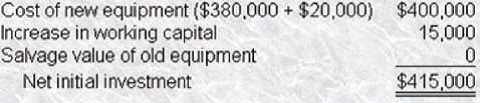Discussions

##### Question 3

The management of Pelican, Inc. is evaluating a proposed acquisition of a new machine at a purchase
price of \$180,000 and with installation costs of \$10,000. A \$9,000 increase in working capital will be
required. The machine Will have a useful life of four years, after which it can be sold for \$30,000. The
estimated annual incremental operating revenues and cash operating expenses are \$450,000 and
\$300.000, respectively, for each of the four years. Pelicans effective income tax rate is 40%. and the
cost of capital is 12%. Pelican uses straight-line depreciation for both financial reporting and income
tax purposes. If the project is accepted, the estimated incremental after-tax operating cash flows at
the end of the first year wilt be?

• A. \$99,000 652/654 Questions & Answers PDF P-
• B. \$106,000
• C. \$108,000
• D. \$150,000

B

Explanation:
The estimated incremental after-tax operating cash flows for each year of a capital project consist of
two components: the after-tax cash inflows from operations and the depreciation tax shield arising
from me purchase of new equipment. The first of these for Pelican can be calculated as follows: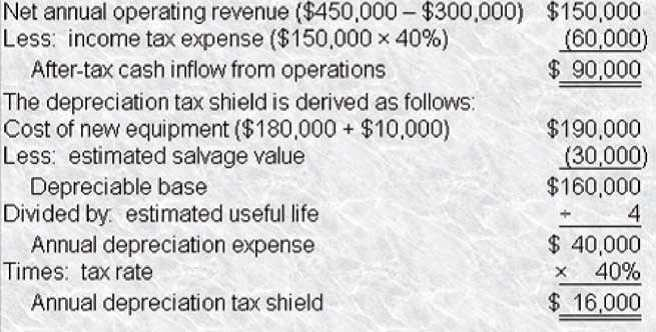Pelicans total incremental after-tax operating cash flows for each year of the projects
life is thus \$106,000 (\$90,000 + \$16,000).

Discussions

##### Question 4

The chief financial officer of Pauley, Inc has requested an evaluation of a proposed acquisition of a
new machine at a purchase price of \$60.000 and with installation costs of \$10,000. A \$3,000 increase
in working capital will be required. The machine will have a useful life of four years. after which it can
be sold for \$10,000. The estimated annual incremental operating revenues and cash operating
expenses are \$150,000 and \$100,000, respectively, for each of the four years. Pauleys effective
income tax rate is 40%, and the cost of capital is 12%. Pauley uses straight-line depreciation for both
financial reporting and income tax purposes. Pauleys estimated after-tax cash flow in the fourth
year, at which time the equipment will be sold, will be?

• A. \$34,000
• B. \$45,000
• C. \$46,000 D \$49,000

D

Explanation:
The estimated incremental after-tax operating cash flows for each year of a capital project consist of
651/654
P-
two components: the after-tax cash inflows from operations and the depreciation tax shield arising
from the purchase of new equipment. The first of these for Pauley can be calculated as follows: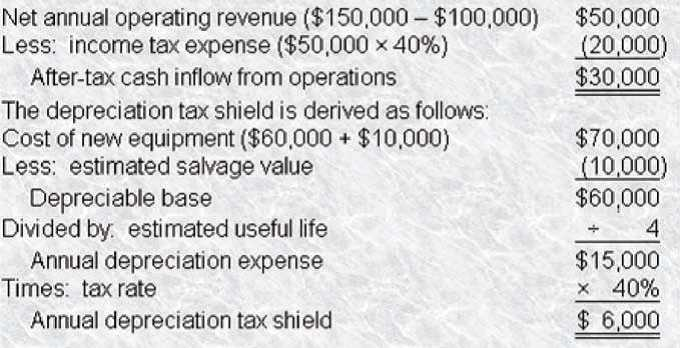Pauleys total after-tax operating cash inflow for each year of the projects life is thus \$36,000
(\$30,000 + \$6,000). Ii the final year of the project, two additional cash flows must be taken into
account, the after-tax proceeds from the disposal of the equipment purchased for the project, and
the recovery of working capital devoted to the project. These two additional cash flows can be
calculated as follows: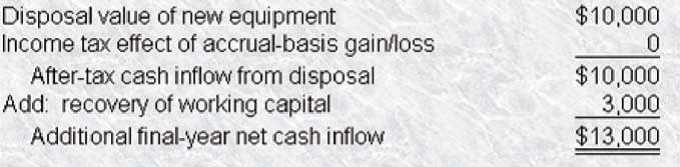Pauleys total after-tax cash inflow for the final year of the projects life is thus \$49,000
(\$36,000 + \$13,000).

Discussions

##### Question 5

The Hopkins Company has estimated that a proposed projects 10-year annual net cash benefit,
received each year end. will be \$2,500 with an additional terminal benefit of \$5,000 at the end of the
10th year. Assuming that these cash inflows satisfy exactly Hopkins required rate of return of 8%,
calculate the initial cash outlay

• A. \$16,775 B \$19,090
• C. \$25,000
• D. \$30,000

B

Explanation:
If the 8% return exactly equals the present value of the future flows ., NPV is zero), then simply
determine the present value of the future inflows. Thus, Hopkins Companys initial cash outlay is
\$19,090 [(\$2,500)(PVIFA at 8% for 10 periods) + (\$5J00)(PVlF at 8% for 10 periods (\$2,500)(6.710) +
(\$5,000)(.463)].

Discussions

##### Question 6

Union Electric Company must clean up the water released from its generating plant. The companys
cost of capital is 12 percent for average risk projects, and that rate is normally adjusted up or down
by 2 percentage points for high- and low- risk projects. Clean-Up Plan

• A. \$15,432,000
• B. \$15,650,000
• C. \$16,300,000
• D. \$17,290,000

B

Explanation:
The cash flows of Plan A are discounted at 12%, the companys cost of capital for average risk
projects. Plan B is evaluated with a lower cost of capital that reflects a greater risk of the cash
outflow of the project. Thus, the cash flows of Plan B are discounted at 10% (12% 2%). the
companys adjusted cost of capital for high risk projects. The net present value of each plan is the
initial cost plus the present value of an annuity for 10 years at the appropriate rate multiplied times
the annual operating cost.
650/654
P-
The present value factors are found in the tools section of CMA Test Prep.
Plan A NPV = \$10,000,000 + (\$1,000,000 x 5.650)
Plan A NPV = \$15,650,000
Plan B NPV = \$5,000,000 + (\$2,000,000 x 6.145)
Plan B NPV = \$17,290,000
Plan A has a lower NPV and thus is the better project.

Discussions

##### Question 7

The U.S. Postal Service is looking for a new machine to help sort the mail. Two companies have
submitted bids to Cliff Kraven, the postal inspector responsible for choosing a machine. A cash flow
analysis of the two machines indicates the following: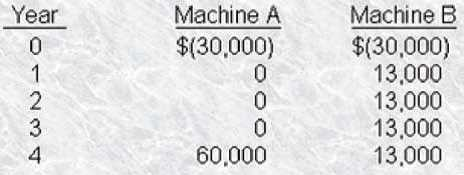It the cost of capital for the Postal Service is 8%. which of the two mail sorters should Cliff choose and
why?

• A. Machine A. because NPV of A > NPV of B. by \$1,044. 649/654 Questions & Answers PDF P-
• B. Machine B. because NPV of A > NPV of B. by \$22,000.
• C. Machine A. because NPV of A > NPV of B. by \$8,000
• D. Machine B. because IRR of A > IRR of B.

A

Explanation:
The NPV of both machines must be calculated and compared to determine which will yield a better
return of cash flows. Machine A is calculated as one lump sum payable in 4 years minus the initial
investment cost.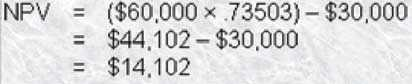The NPV of Machine B is calculated as the present value of an ordinary annuity of
\$13,000 for 4 years, minus the initial investment cost.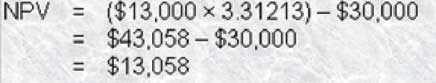By comparing the NPV of both machines, Cliff would choose Machine A because NPV of A > NPV of B
by \$1,044.

Discussions

##### Question 8

The tax impact of equipment depreciation affects capital budgeting decisions. Currently, me
Modified Accelerated Cost Recover System (MACRS) is used as the depreciation method for most
assets for tax purposes. When employing the MACRS method of depreciation in a capita) budgeting
decision, the use of MACRS as compared with the straight-line method of depreciation will result in?

• A. Equal total depreciation for both methods.
• B. MACRS producing less total depreciation than straight line.
• C. Equal total tax payments, after discounting for the time value of money. D MACRS producing more total depreciation than straight line

A

Explanation:
For tax purposes. straight-line depreciation is an alternative to the MACRS method. Both methods
will result in the same total depreciation over the life of the asset: however, MACRS will result in
greater depreciation in the early years of the assets life because it is an accelerated method. Given
that MACRS results in larger depreciation deductions in the earj years, taxes will be lower in the early
years and higher in the later years. Because the incremental benefits will be discounted over a
shorter period than the incremental depreciation costs. MACRS is preferable to the straight-line
method,

Discussions

##### Question 9

The tax impact of equipment depreciation affects capital budgeting decisions. Currently, the
Modified Accelerated Cost Recovery System (MACRS) is used as the depreciation method for most
assets for tax purposes. The MACRS method of depreciation for assets with 3, 5. 7. and 1 0-year
recovery periods is most similar to which one of the following depreciation methods used for
financial reporting purposes?
A. Straight-line.
B Units-of-production.
C. Sum-of-the-years-digits.
D. 200% declining-balance.

D
648/654
P-

Explanation:
MACRS for assets with lives of 10 years or less is based on the 200% declining-balance method of
depreciation. Thus, an asset with a 3-year life would have a straight-line rate of 33-113%. or a
double- declining-balance rate of 66-2/3%.

Discussions

##### Question 10

Capital budgeting is used for the decision analysis of?

• A. Adding product lines or facilities.
• B. Multiple profitable alternatives.